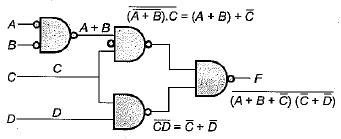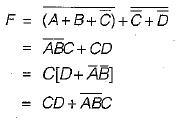Courses

# Combinational Circuit (Basic Level) - 1

## 10 Questions MCQ Test Question Bank for GATE Computer Science Engineering | Combinational Circuit (Basic Level) - 1

Description
This mock test of Combinational Circuit (Basic Level) - 1 for Computer Science Engineering (CSE) helps you for every Computer Science Engineering (CSE) entrance exam. This contains 10 Multiple Choice Questions for Computer Science Engineering (CSE) Combinational Circuit (Basic Level) - 1 (mcq) to study with solutions a complete question bank. The solved questions answers in this Combinational Circuit (Basic Level) - 1 quiz give you a good mix of easy questions and tough questions. Computer Science Engineering (CSE) students definitely take this Combinational Circuit (Basic Level) - 1 exercise for a better result in the exam. You can find other Combinational Circuit (Basic Level) - 1 extra questions, long questions & short questions for Computer Science Engineering (CSE) on EduRev as well by searching above.
QUESTION: 1

Solution:
QUESTION: 2

### Which expression is computed by the following NAND-gate circuit diagram:Solution: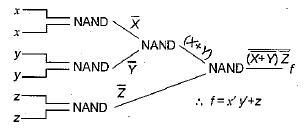QUESTION: 3

### What is the Boolean expression for the following circuit: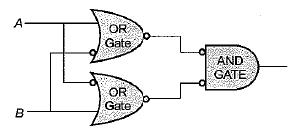Solution: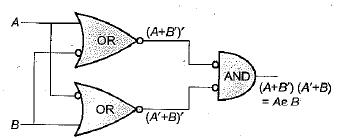QUESTION: 4

A multiplexer is also known as

Solution:
QUESTION: 5

The quantity 837 in excess-3 BCD code would be represented as

Solution:

The quantity 837 is first converted to BCD representation then it is incremented by 3 bit by bit.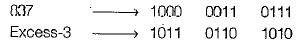QUESTION: 6

Solution:
QUESTION: 7

A comparison between serial and parallel adder reveals that serial order

Solution:
QUESTION: 8

How many full adders are needed to construct an m-bit parallel adder ;

Solution:

We can construct a m-bit parallel adder by,
iii) (2m - 1 ) full adders and (n - 1) OR GATES

QUESTION: 9

Identify the logic function performed by the circuit shown in the given figure: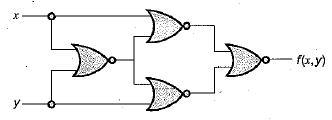Solution: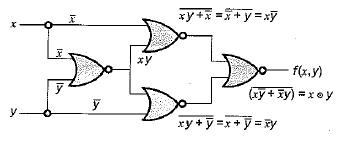Therefore the above circuit performed exclusive NOR gate.

QUESTION: 10

The logic expression for the output of the circuit shown in the figure below is: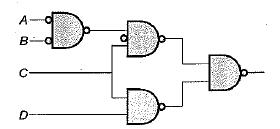Solution: